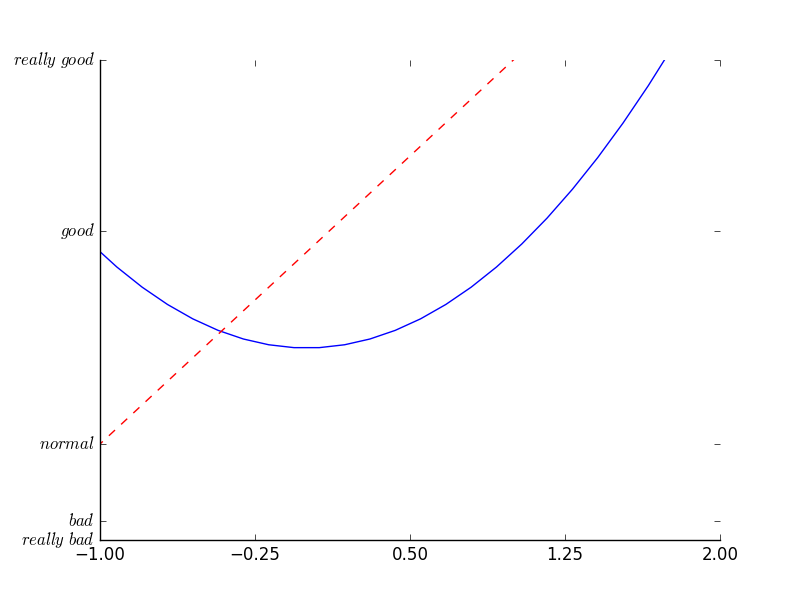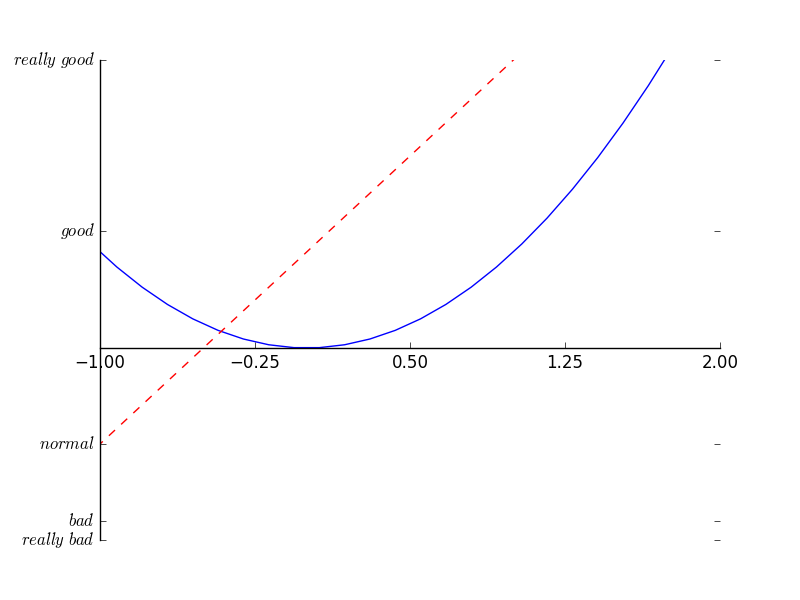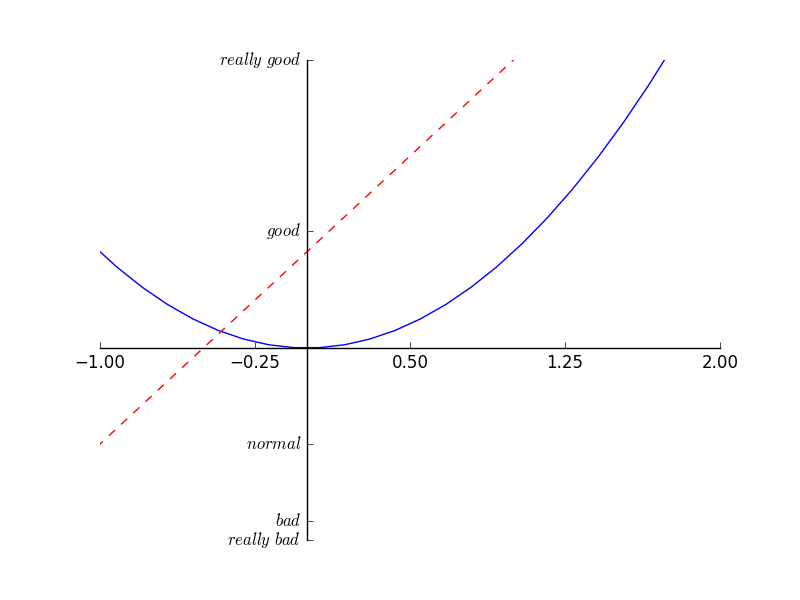# matplotlib 设置坐标轴2

378℃

## 设置不同名字和位置

``````import matplotlib.pyplot as plt
import numpy as np``````

``````x = np.linspace(-3, 3, 50)
y1 = 2*x + 1
y2 = x**2``````

``````plt.figure()
plt.plot(x, y2)
plt.plot(x, y1, color='red', linewidth=1.0, linestyle='--')
plt.xlim((-1, 2))
plt.ylim((-2, 3))``````

``````new_ticks = np.linspace(-1, 2, 5)
plt.xticks(new_ticks)

``````ax = plt.gca()
ax.spines['right'].set_color('none')
ax.spines['top'].set_color('none')
plt.show()``````## 调整坐标轴

``ax.xaxis.set_ticks_position('bottom')``

``````ax.spines['bottom'].set_position(('data', 0))
plt.show()````````ax.yaxis.set_ticks_position('left')``

``````ax.spines['left'].set_position(('data',0))
plt.show()``````